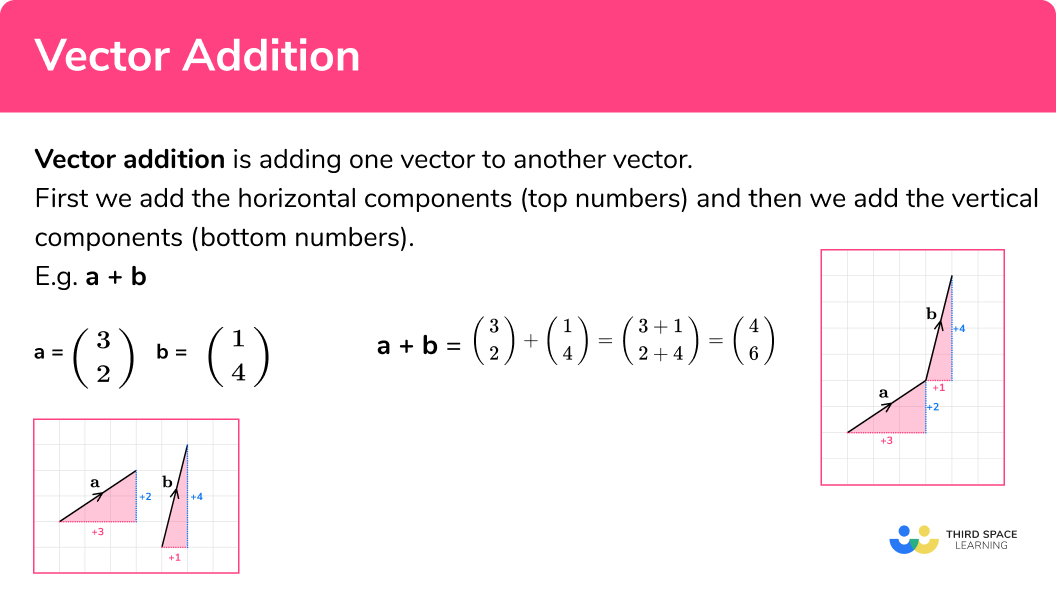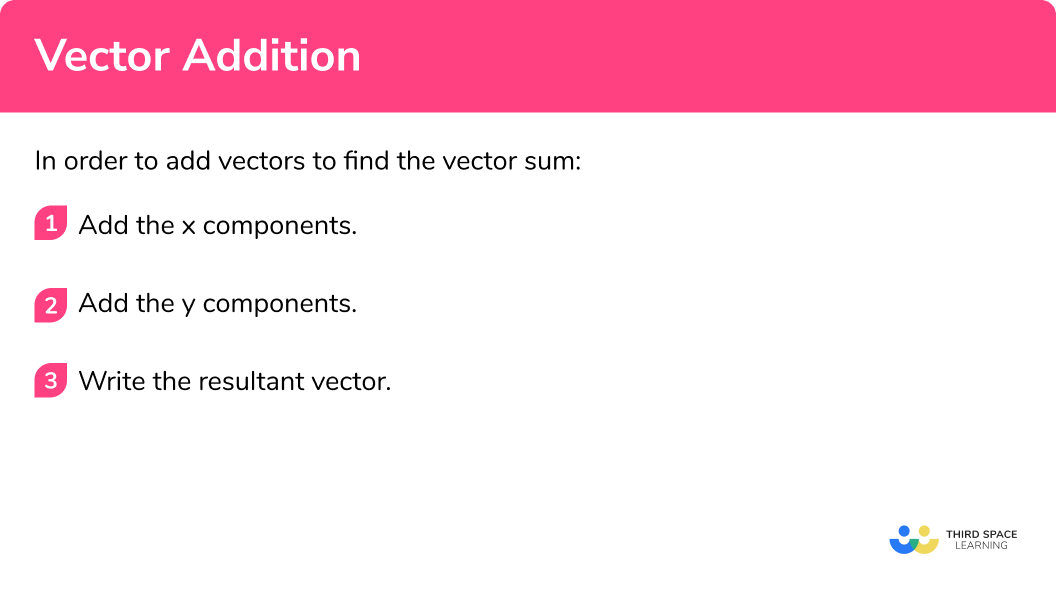There are also vector worksheets based on Edexcel, AQA and OCR exam questions, along with further guidance on where to go next if you’re still stuck.

Vector addition is adding one vector to another vector. This is sometimes known as a vector sum.

To do this we add the individual components of the first vector to the second vector.
First we add the horizontal components of a vector (top numbers) and then we add the vertical components of a vector (bottom numbers).

E.g.

Let’s look at some examples of vectors, vector a and vector b.

$\textbf{a}= \left(\begin{array}{1} 3\\ 2\\ \end{array}\right) \quad \textbf{b}= \left(\begin{array}{1} 1\\ 4\\ \end{array}\right)$

Here are the vectors represented diagrammatically:

For the addition of vectors, a + b, we add vector b onto the end of vector a.

$\textbf{a} +\textbf{b}= \left(\begin{array}{1} 3\\ 2\\ \end{array}\right) + \left(\begin{array}{1} 1\\ 4\\ \end{array}\right)$

$\textbf{a} +\textbf{b}= \left(\begin{array}{1} 3\\ 2\\ \end{array}\right) + \left(\begin{array}{1} 1\\ 4\\ \end{array}\right) = \left(\begin{array}{1} 4\\ 6\\ \end{array}\right)$

When finding a vector sum we do not need to draw a diagram. We can just add the vector components. First, add the x components (top numbers) and then add the y components (bottom numbers)

$\textbf{a} +\textbf{b} = \left(\begin{array}{1} 3\\ 2\\ \end{array}\right) +\ \left(\begin{array}{1} 1\\ 4\\ \end{array}\right) = \left(\begin{array}{1} 3+1\\ 2+4\\ \end{array}\right) = \left(\begin{array}{1} 4\\ 6\\ \end{array}\right)$

The new vector that results from adding two given vectors is known as the resultant vector.

The addition of vectors is commutative which means that the order in which we add the vectors is not important. In other words we will get the same result if we start with vector b and add vector a.

\textbf{a} +\textbf{b}= \textbf{b} +\textbf{a}

The diagram is a parallelogram. The resultant vector is the diagonal of the parallelogram.In order to add vectors to find the vector sum:

3. Write the resultant vector.## Related lessons on vectors

Vector addition is part of our series of lessons to support revision on vectors. You may find it helpful to start with the main vectors lesson for a summary of what to expect, or use the step by step guides below for further detail on individual topics. Other lessons in this series include:

$\left(\begin{array}{1} 2\\ 4\\ \end{array}\right) \text{and}\ \left(\begin{array}{1} 5\\ 1\\ \end{array}\right)$

Add the two top numbers of the original vectors

2+5=7

4+1=5

3Write the resultant vector.

$\left(\begin{array}{1} 2\\ 4\\ \end{array}\right) + \left(\begin{array}{1} 5\\ 1\\ \end{array}\right) =\ \left(\begin{array}{1} 7\\ 5\\ \end{array}\right)$

$\left(\begin{array}{1} 4\\ 2\\ \end{array}\right) \text{and}\ \left(\begin{array}{1} 1\\ 7\\ \end{array}\right)$

Write the resultant vector.

$\left(\begin{array}{1} 4\\ 1\\ \end{array}\right) \text{and}\ \left(\begin{array}{1} -2\\ 3\\ \end{array}\right)$

Write the resultant vector.

$\left(\begin{array}{1} -5\\ 2\\ \end{array}\right) \text{and}\ \left(\begin{array}{1} 2\\ -4\\ \end{array}\right)$

Write the resultant vector.

$\left(\begin{array}{1} 7\\ 3\\ \end{array}\right) \text{and}\ \left(\begin{array}{1} -3\\ -4\\ \end{array}\right)$

Write the resultant vector.

$\left(\begin{array}{1} -3\\ -1\\ \end{array}\right) \text{and}\ \left(\begin{array}{1} -2\\ 4\\ \end{array}\right)$

Write the resultant vector.

### Common misconceptions

Mistakes can easily be made when adding a positive number and a negative number.

Make sure that negative numbers skills are well practiced.

E.g.

3+-4=3-4=-1

• Column vectors notation

Column vectors only have 2 numbers within the brackets, they have the top number and the bottom number.

There is no need for any other punctuation marks such as commas or semicolons and there is no need for a line to separate the numbers.

1. Work out:

\begin{pmatrix} \; 3 \;\\ \; 2 \; \end{pmatrix} + \begin{pmatrix} \; 1 \;\\ \; 5 \; \end{pmatrix}

\begin{pmatrix} \; 3 \;\\ \; 10 \; \end{pmatrix}\begin{pmatrix} \; 2 \;\\ \; -3 \; \end{pmatrix}\begin{pmatrix} \; 4 \;\\ \; 7 \; \end{pmatrix}\begin{pmatrix} \; 4 \;\\ \; -3 \; \end{pmatrix}\begin{pmatrix} \; 3 \;\\ \; 2 \; \end{pmatrix} + \begin{pmatrix} \; 1 \;\\ \; 5 \; \end{pmatrix} = \begin{pmatrix} \; 3+1 \;\\ \; 2+5 \; \end{pmatrix} = \begin{pmatrix} \; 4 \;\\ \; 7 \; \end{pmatrix}

2. Work out:

\begin{pmatrix} \; 4 \;\\ \; 5 \; \end{pmatrix} + \begin{pmatrix} \; 1 \;\\ \; -3 \; \end{pmatrix}

\begin{pmatrix} \; 5 \;\\ \; 8 \; \end{pmatrix}\begin{pmatrix} \; 4 \;\\ \; -15 \; \end{pmatrix}\begin{pmatrix} \; 4 \;\\ \; 2 \; \end{pmatrix}\begin{pmatrix} \; 5 \;\\ \; 2 \; \end{pmatrix}\begin{pmatrix} \; 4 \;\\ \; 5 \; \end{pmatrix} + \begin{pmatrix} \; 1 \;\\ \; -3 \; \end{pmatrix} = \begin{pmatrix} \; 4+1 \;\\ \; 5+-3 \; \end{pmatrix} = \begin{pmatrix} \; 5 \;\\ \; 2 \; \end{pmatrix}

3. Work out:

\begin{pmatrix} \; -2 \;\\ \; 8 \; \end{pmatrix} + \begin{pmatrix} \; -1 \;\\ \; 3 \; \end{pmatrix}

\begin{pmatrix} \; 3 \;\\ \; 11 \; \end{pmatrix}\begin{pmatrix} \; -3 \;\\ \; 11 \; \end{pmatrix}\begin{pmatrix} \; 3 \;\\ \; 5 \; \end{pmatrix}\begin{pmatrix} \; -3 \;\\ \; -11 \; \end{pmatrix}\begin{pmatrix} \; -2 \;\\ \; 8 \; \end{pmatrix} + \begin{pmatrix} \; -1 \;\\ \; 3 \; \end{pmatrix} = \begin{pmatrix} \; -2+-1 \;\\ \; 8+3 \; \end{pmatrix} = \begin{pmatrix} \; -3 \;\\ \; 11 \; \end{pmatrix}

4. Work out:

\begin{pmatrix} \; -2 \;\\ \; 3 \; \end{pmatrix} + \begin{pmatrix} \; 4 \;\\ \; -7 \; \end{pmatrix}

\begin{pmatrix} \; 2 \;\\ \; -4 \; \end{pmatrix}\begin{pmatrix} \; 2 \;\\ \; 4 \; \end{pmatrix}\begin{pmatrix} \; -2 \;\\ \; -4 \; \end{pmatrix}\begin{pmatrix} \; -2 \;\\ \; 4 \; \end{pmatrix}\begin{pmatrix} \; -2 \;\\ \; 3 \; \end{pmatrix} + \begin{pmatrix} \; 4 \;\\ \; -7 \; \end{pmatrix} = \begin{pmatrix} \; -2+4 \;\\ \; 3+-7 \; \end{pmatrix} = \begin{pmatrix} \; 2 \;\\ \; -4 \; \end{pmatrix}

5. Work out:

\begin{pmatrix} \; -3 \;\\ \; 6 \; \end{pmatrix} + \begin{pmatrix} \; -2 \;\\ \; 2 \; \end{pmatrix}

\begin{pmatrix} \; 5 \;\\ \; 8 \; \end{pmatrix}\begin{pmatrix} \; 6 \;\\ \; 12 \; \end{pmatrix}\begin{pmatrix} \; 6 \;\\ \; 8 \; \end{pmatrix}\begin{pmatrix} \; -5 \;\\ \; 8 \; \end{pmatrix}\begin{pmatrix} \; -3 \;\\ \; 6 \; \end{pmatrix} + \begin{pmatrix} \; -2 \;\\ \; 2 \; \end{pmatrix} = \begin{pmatrix} \; -3+-2 \;\\ \; 6+2 \; \end{pmatrix} = \begin{pmatrix} \; -5 \;\\ \; 8 \; \end{pmatrix}

6. Work out:

\begin{pmatrix} \; -4 \;\\ \; -1 \; \end{pmatrix} + \begin{pmatrix} \; -3 \;\\ \; 4 \; \end{pmatrix}

\begin{pmatrix} \; -7 \;\\ \; -3 \; \end{pmatrix}\begin{pmatrix} \; 7 \;\\ \; -3 \; \end{pmatrix}\begin{pmatrix} \; -7 \;\\ \; 3 \; \end{pmatrix}\begin{pmatrix} \; 7 \;\\ \; 3 \; \end{pmatrix}\begin{pmatrix} \; -4 \;\\ \; -1 \; \end{pmatrix} + \begin{pmatrix} \; -3 \;\\ \; 4 \; \end{pmatrix} = \begin{pmatrix} \; -4+-3 \;\\ \; -1+4 \; \end{pmatrix} = \begin{pmatrix} \; -7 \;\\ \; 3 \; \end{pmatrix}

1. Which of the vectors below is the sum of

\begin{pmatrix} \; 5 \;\\ \; 3 \; \end{pmatrix} \; and \; \begin{pmatrix} \; 2 \;\\ \; -5 \; \end{pmatrix} ?

(1 mark)

C

(1)

2. Here are two vectors.

\textbf{a}= \begin{pmatrix} \; -3 \;\\ \; 4 \; \end{pmatrix} \; and \; \textbf{b}= \begin{pmatrix} \; 2 \;\\ \; 1 \; \end{pmatrix}

Calculate \textbf{a}+\textbf{b}

(2 marks)

\begin{pmatrix} \; -1 \;\\ \; 5 \; \end{pmatrix}

For the x component (top number)

(1)

For the y component (bottom number)

(1)

3. Here is a vector sum.

\begin{pmatrix} \; \text{a} \;\\ \; 3 \; \end{pmatrix} + \begin{pmatrix} \; 2 \;\\ \; \text{b} \; \end{pmatrix} = \begin{pmatrix} \; 7 \;\\ \; -1 \; \end{pmatrix}

(a) Find the value of a .

(b) Find the value of b .

(2 marks)

(a)

a=5

(1)

(b)

b=-4

(1)

## Learning checklist

You have now learned how to: